# Integral curve

(diff) ← Older revision | Latest revision (diff) | Newer revision → (diff)

The graph of a solutionof a normal system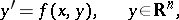of ordinary differential equations. For example, the integral curves of the equation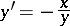are the circles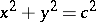, whereis an arbitrary constant. The integral curve is often identified with the solution. The geometric meaning of the integral curves of a scalar equation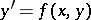(*)

is the following. The equation (*) defines a direction field on the plane, that is, a field of direction vectors such that at each point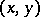the tangent of the angle of inclination of the vector with the-axis is equal to. The integral curves of (*) are then the curves that at each point have a tangent coinciding with the vector of the direction field at this point. The integral curves of (*) fill out the entire region in which the functionsatisfies conditions ensuring the existence and uniqueness of the Cauchy problem; the curves nowhere intersect one another and are nowhere tangent to one another.

How to Cite This Entry:
Integral curve. Encyclopedia of Mathematics. URL: http://encyclopediaofmath.org/index.php?title=Integral_curve&oldid=17766
This article was adapted from an original article by N.N. Ladis (originator), which appeared in Encyclopedia of Mathematics - ISBN 1402006098. See original article